Get instant live expert help with Excel or Google Sheets“My Excelchat expert helped me in less than 20 minutes, saving me what would have been 5 hours of work!”

#### Post your problem and you’ll get Expert help in seconds.

Your message must be at least 40 characters
Our professional Expert are available now. Your privacy is guaranteed.

# How to Get the First Non-blank Value and Text Value in a List in Excel

We can find the First Non-Blank Value (text or number) in a list by applying the INDEX, MATCH and ISBLANK functions. We will also use the ISTEXT function instead of the ISBLANK function to find the First Text Value in our list. We will follow the simple steps below to achieve our objective.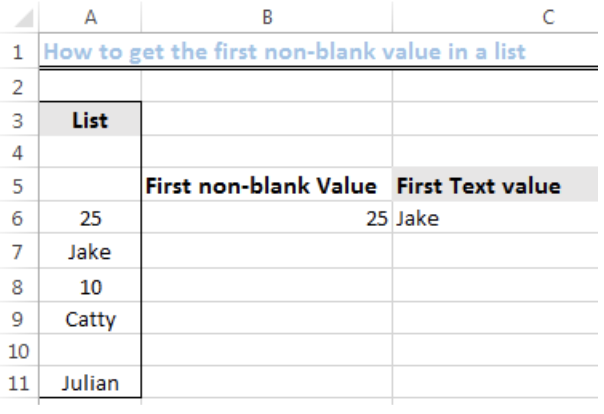Figure 1: Results for First Non-blank Value and Text Value in a List

## Setting up the Data

• We will set up our data by inputting the numeric and text values into Column A, titled, LIST.
• We will type FIRST NON-BLANK VALUE and FIRST TEXT VALUE into Cell B5 and Cell C5 respectively. Refer to figure 1.

## How to get the First Non-blank Value in a List

### Syntax:

` =INDEX(range,MATCH(FALSE,ISBLANK(range),0))`

For us to get the first non-blank value in our list, we will do the following:

• We will click on Cell B6
• We will type or copy and paste the formula below into the cell

`=INDEX(A4:A11,MATCH(FALSE,ISBLANK(A4:A11),0))`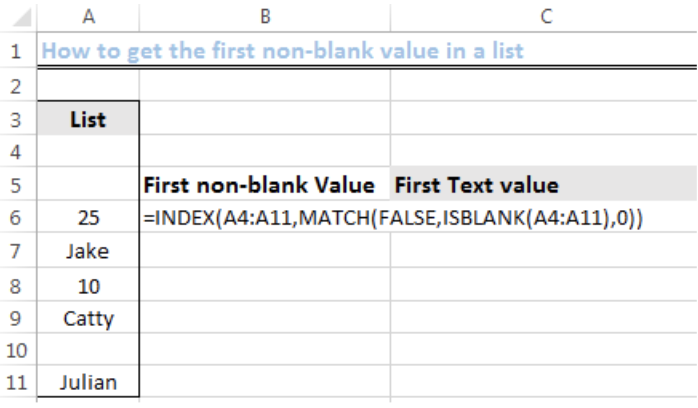Figure 2: How to Find the First Non-blank Value in a List

Once we have inputted the formula, we will press CTRL + SHIFT + ENTER. We will have the result as shown in figure 3.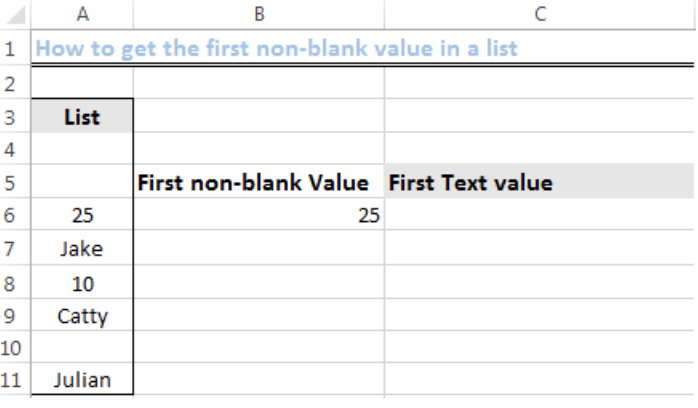Figure 3: Result for the First Non-blank Value in a List

### Explanation of the formula

• `ISBLANK(A4:A11)`

The ISBLANK function tests the cells in the range A4:A11 if there are blank cells or not. It then returns an array that looks like this:

`{TRUE;TRUE;FALSE;FALSE;FALSE;FALSE;TRUE;FALSE}`

TRUE means that a cell is blank. FALSE means that a cell is not blank.

• `MATCH(FALSE,ISBLANK(A4:A11),0)`

MATCH function finds the first FALSE value in the above array (A4:A11) and returns the CELL POSITION of that FALSE value in the array. In this case, the Cell Position in the array is Cell A6.

• `INDEX(A4:A11,MATCH(…))`

Once the first non-blank cell is found, INDEX function returns its value AS TRUE WHETHER IT IS A TEXT OR NUMERIC VALUE.

## How to get the First Text Value in a List

### Syntax:

`=INDEX(range,MATCH(TRUE,ISTEXT(range),0))`

For us to get the first text value in our list, we will do the following:

• We will click on Cell C6
• We will type or copy and paste the formula below into the cell `=INDEX(A4:A11,MATCH(TRUE,ISTEXT(A4:A11),0))`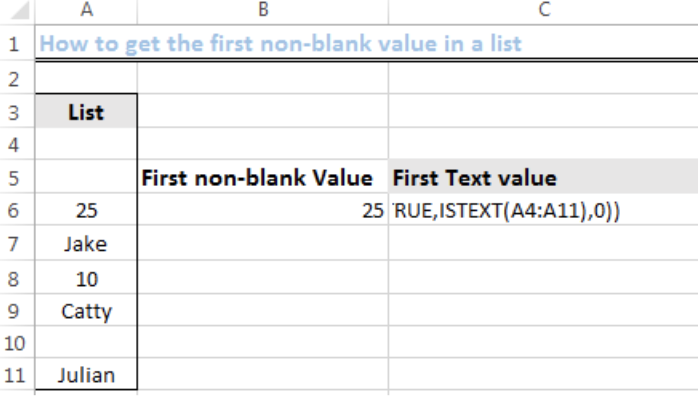Figure 4: How to Find the First Text Value in a List

Once we have inputted the formula, we will press CTRL + SHIFT + ENTER. We will have the result as shown in figure 5.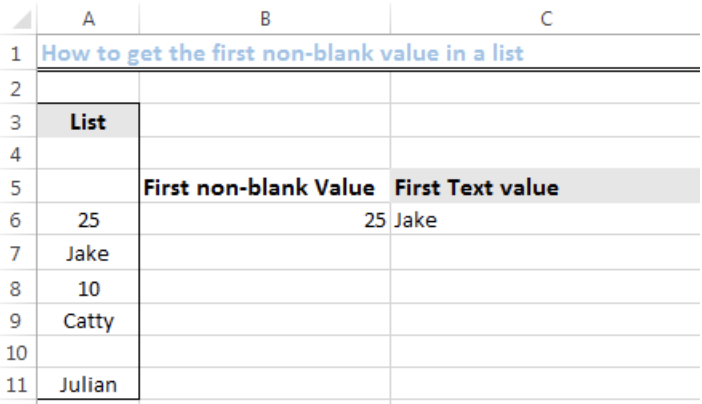Figure 5: Result for the First Text Value in a List

## Explanation of the formula

• `ISTEXT(A4:A11)`

The ISTEXT function tests the cells in the range A4:A11 if there are cells that contain text or not. It then returns an array that looks like this:

`{FALSE; FALSE;FALSE;TRUE;FALSE; TRUE;FALSE;TRUE}`

TRUE means that a cell CONTAINS A TEXT VALUE. FALSE means that a cell doesn’t contain a text value.

• `MATCH(TRUE,ISTEXT(A4:A11),0)`

MATCH function finds the first TRUE value in the above array (A4:A11) and returns the CELL POSITION of that TRUE value in the array. In this case, the Cell Position in the array is Cell A7.

• `INDEX(A4:A11,MATCH(…))`

Once the first TEXT-CONTAINING CELL is found, INDEX function returns its value AS TRUE.

## Instant Connection to an Expert through our Excelchat Service:

Most of the time, the problem you will need to solve will be more complex than a simple application of a formula or function. If you want to save hours of research and frustration, try our live Excelchat service! Our Excel Experts are available 24/7 to answer any Excel question you may have. We guarantee a connection within 30 seconds and a customized solution within 20 minutes.

### Did this post not answer your question? Get a solution from connecting with the expert.Another blog reader asked this question today on Excelchat:
Solution examplesI need a formula to compare the data in two columns and then export the mismatched data in the 3rd column
Solved by S. Q. in 20 minsI need to sum the number of hours per month one of three halls are being rented
Solved by A. F. in 60 minsI have a list or people who are ranked in numbers from 3 to 6 I need to recognize the contents of each multiple cells and create an equivalent letter. for that value into another column. e.g. Cell E1 = 3 to show in new cell that row (H1) the letter "C" I have multiple rows with different values in column "E" Can this be done?
Solved by I. J. in 30 minsI have a cell that has a working vlook formula in it. When it looks up source data it returns blank. That is fine. I now want to use an IF formula to say if that cell is blank leave the other cell blank and if not then show value in different cell. The issue I have is that when the IF formula looks up the cell it reports back FALSE due to the vlook up formula being in the cell. I want the IF formula to show nothing instead of FALSE. Can you help?
Solved by Z. E. in 60 minsI need a formula that will check if 2 or more cells containing names match then add the adjacent cells to matching name together.
Solved by F. D. in 59 mins## Subscribe to Excelchat.coAnother blog reader asked this question today on Excelchat: